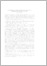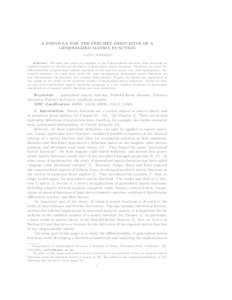# A Formula for the Fréchet Derivative of a Generalized Matrix Function

Noferini, Vanni (2017) 'A Formula for the Fréchet Derivative of a Generalized Matrix Function.' SIAM Journal on Matrix Analysis and Applications, 38 (2). 434 - 457. ISSN 0895-4798Preview
Text
gendk_SIMAX_rev2c.pdf - Accepted Version

Download (187kB) | Preview

## Abstract

We state and prove an analogue of the Daleckii--Krein theorem, thus obtaining an explicit formula for the Fréchet derivative of generalized matrix functions. Moreover, we prove the differentiability of generalized matrix functions of real matrices under very mild assumptions. For complex matrices, we argue that, under the same assumptions, generalized matrix functions are real-differentiable but generally not complex-differentiable. Finally, we discuss the application of our results to the study of the condition number of generalized matrix functions. Along our way, we also derive generalized matrix functional analogues of a few classical theorems on polynomial interpolation of classical matrix functions and their derivatives. Read More: https://epubs.siam.org/doi/10.1137/16M1072851

Item Type: Article generalized matrix function, Daleckii--Krein theorem, Gâteaux derivative, Fréchet derivative, condition number Q Science > QA Mathematics Faculty of Science and Health > Mathematical Sciences, Department of Vanni Noferini 19 Jul 2017 11:09 29 Jun 2018 12:15 http://repository.essex.ac.uk/id/eprint/20099

### Actions (login required)View Item